In mathematics, we use letters to perform calculations. This is called an algebraic expression. Before learning the algebraic expression, calculations are done using only numbers. However, after learning algebraic expressions, math is mainly done by using the alphabet.

Algebraic expressions have rules. Therefore, you have to learn how to use algebraic expressions.

There are also several types of equations that are represented by symbols: monomials and polynomials. What are the differences between these types of expressions? If you don’t understand the rules of algebraic expressions, you can’t add or subtract using these expressions.

So, we’ll explain the rules of algebraic expressions, the difference between monomial and polynomial formulas, and how to add and subtract using these expressions.

## In an Algebraic Expression, Turning Unknown Numbers into Symbols

What kind of calculation is the algebraic expression that we learn in math? In an algebraic expression, we use letters of the alphabet to represent equations as follows.

• $10+a$
• $2x+y$
• $2a^2b$

When we look at equations that contain symbols, you may think that it looks difficult to understand.

However, we have been studying algebraic expressions in elementary school. For example, what is included in the following $□$?

• $10-□=4$

You must have solved a problem like this before. The number that goes in □ is 6. For numbers we don’t know, we make an equation by using □. Instead, in the algebraic expressions, the unknown numbers are represented by the alphabet.

For example, in the previous problem, we can replace $□$ with $x$ and write the following.

• $10-x=4$

This is an algebraic expression. We use letters, such as $x, y, a, b$, etc., to represent numbers we don’t know and create an equation.

-Algebraic Expressions Are Useful in Calculations

Why do we use algebraic expressions? It is because they are useful in math calculations. Mathematics is used frequently in our daily life. In most cases, we already know the answer, but we don’t know how to express the process.

For example, here’s an example.

• How many kilometers should you walk per day to walk 1,000 km in 365 days (one year)?

We already know the answer to walking 1,000 km. But we don’t know the process of how many kilometers a day we should walk. So, we replace the numbers we don’t know with symbols such as $x$ and calculate them.

Even if we don’t know the numbers, we can construct an equation and do the calculation. This is the reason why we use algebraic expressions in mathematics.

### Understand the Rules of Algebraic Expressions

What are the rules in algebraic expressions? Be sure to follow the following rules in algebraic expressions.

• Omit the × in multiplication.
• Write numbers first, then alphabetically.
• Use exponents to multiply the same letter.
• Omit 1 and -1.
• Don’t use division; use multiplying fractions.

The rules are not difficult to understand. Just make sure you understand them.

-Omit the × in Multiplication.

When multiplying, we always use the symbol “x.” However, in algebraic expressions, the × is omitted when multiplying. Therefore, you get the following.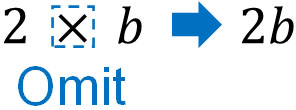Instead of $2×b$, write $2b$. Also, write $ab$ instead of $a×b$. In the algebraic expression, the × is omitted.

-Write Numbers First, Then Alphabetically

In the algebraic expression, what order should we write them in? The rule is that numbers should always be written before the alphabet. Also, if there are several letters, they should be written in alphabetical order.

For example, the following multiplication can be expressed by the following algebraic expression.

• $a×4×b=4ab$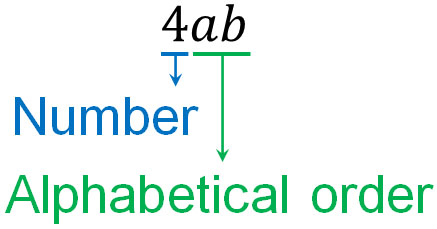The number 4 is written first. The letters are written in alphabetical order, so they are written as $4ab$.

-Use Exponents to Multiply the Same Letter

In algebraic expressions, we frequently multiply the same letter. For example, $a×a$. However, we never write $aa$ in an algebraic expression. In the multiplication of the same letter, we use exponents. For example, we have the following.

• $a×a=a^2$
• $3×y×y×y=3y^3$

-Omit 1 and -1

The rule for writing numbers first makes us think that we have to write them as follows.

• $1×a=1a$
• $-1×b=-1b$

However, we have to omit 1 and -1 because 1 can be multiplied infinitely, and can be expressed as $1×1×1×a$ and so on. To prevent this, we omit the numbers 1 and -1. For -1, we write the $-$, as in $-b$. Thus, we have the following.

• $1×a=a$
• $-1×b=-b$

-Don’t Use Division; Use Multiplying Fractions

We don’t use division in algebraic expressions. Division has limited use, and few people use division in mathematics. In fact, no one uses division in junior high and high school mathematics.

Division can be converted to multiplying fractions. Don’t use division in algebraic expressions, but use equations with multiplication only.

### Learn the Algebraic Expressions for Fractions and Reciprocal Numbers

One of the most difficult concepts to understand when learning algebraic expressions is to convert division into multiplying fractions. So, we’ll explain how to change the division of algebraic expressions into fractions.

Divisions can be converted to multiplying fractions by using the reciprocal, as follows.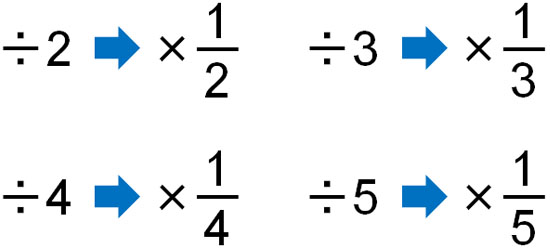The same is true for algebraic expressions. The division of algebraic expressions can be converted to multiplying fractions by using the reciprocal, as shown below.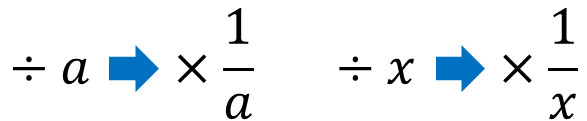Note that in the algebraic expression, you can write the alphabet in the numerator or next to the fraction. So, it looks like this.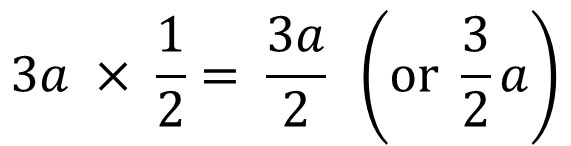Both notations are correct. You can use either, but you should be able to write both.

As a reminder, if there are letters in the denominator, the alphabet must be in the denominator. Do not write the alphabet next to the fraction. Therefore, it looks like this.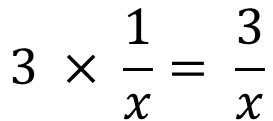As mentioned above, if there are alphabets in the numerator, you can write them in the numerator or next to the fraction. However, if there are alphabets in the denominator, be sure to write them in the denominator.

## The Difference Between Monomials and Polynomials in Algebraic Expressions

After understanding the rules about algebraic expressions, we need to understand the meaning of the words. When we study algebraic expressions, the words we must learn are monomials and polynomials. Both monomials and polynomials are expressions that use letters and numbers.

The differences between them are as follows.

• Monomials: equations that only involve multiplication
• Polynomials: equations that mix addition and subtraction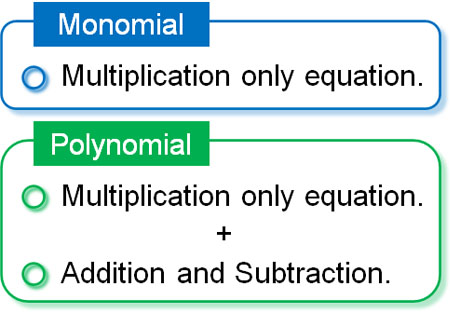You can understand that a monomial is, in essence, a single algebraic expression.

We explained that in an algebraic expression, you omit the × when you multiply. As a result, in an algebraic expression with only multiplication, there will be a sequence of numbers and symbols. In this case, the mass of numbers and letters is a monomial.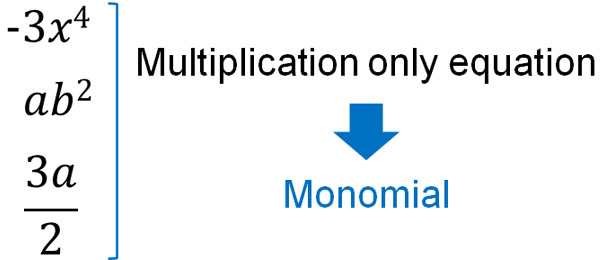However, in mathematics, the equation does not necessarily include only multiplication. While division can be converted to multiplication, addition, and subtraction cannot be converted to multiplication.

So if there are additions and subtractions in an equation, there are several monomials. When an equation contains addition and subtraction, resulting in the existence of multiple monomials, it is called a polynomial.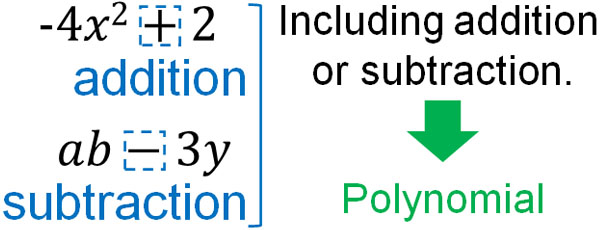The difference between monomials and polynomials is determined by whether addition or subtraction is included in the equation. A polynomial is when there are two or more monomials in the equation, using pluses and minuses.

### Coefficients, Terms, and Degrees of Monomials and Polynomials

For reference, when we learn monomials and polynomials in algebraic expressions, we will see the words coefficient, term, and degree. Each of them is as follows.

• Coefficient: a number in a monomial
• Term: a monomial including numbers and letters
• Degree: numbers of letters, such as $x$ and $a$

You don’t need to understand and remember the definitions of these words. However, these words are always found in textbooks that explain monomials and polynomials, so you should understand roughly what they mean.

Coefficients refer to numbers in monomials. For example, it is as follows.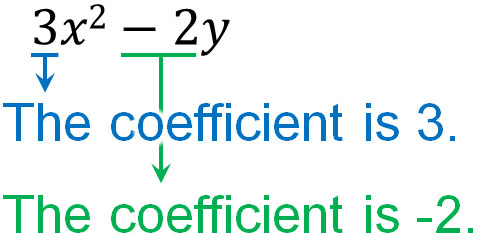Understand that the numbers in front of the letters, including pluses and minuses, are coefficients.

On the other hand, each monomial is called a term. In the previous equation, the following are the terms.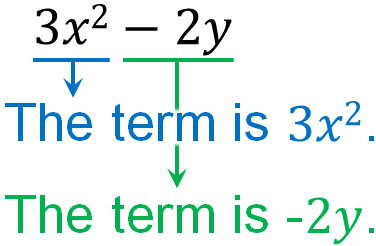It is easy to distinguish between coefficients and terms. However, coefficients and terms are not important words in mathematics. The more important word is degree.

The degree refers to the number of letters (alphabets) contained in a monomial.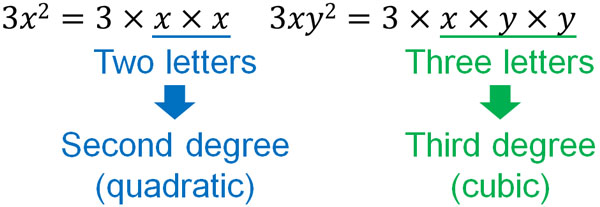• One letter: first degree (linear)
• Two letters: second degree (quadratic)
• Three letters: third degree (cubic)
• Four letters: fourth degree (quartic)

Note that a polynomial contains multiple monomials of different degrees. Among these monomials, the highest number of letters is used as the degree.

It is important to understand the definition of degree in a polynomial. The monomial that contains the highest number of letters is the degree of the polynomial. For example, the following equation contains several monomials.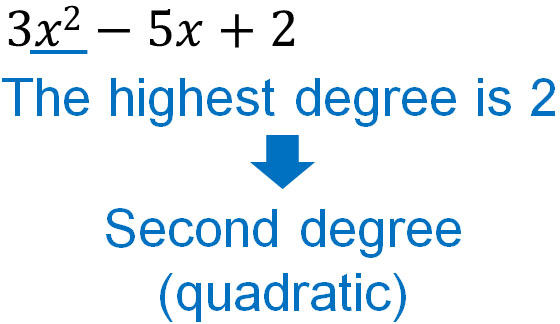In this equation, the largest degree is 2. Therefore, this polynomial is quadratic.

In mathematics, we learn formulas for linear functions and quadratic functions. How do we know if it is a linear function or a quadratic function? You can distinguish them by the degree of the polynomial.

## Addition and Subtraction of Polynomials: Combining Like Terms (Similar Terms)

After learning about monomials and polynomials in algebraic expressions, the next step we need to understand is addition and subtraction. We’ve already learned about addition and subtraction. But how can we do addition and subtraction for algebraic expressions that contain alphabets?

Even though letters, such as $x$ and $y$, are included in the expression, these algebraic expressions are the same as numbers. For example, suppose we have the following equation.

• $3x+1$

You can put any number in $x$. So the answer changes as follows.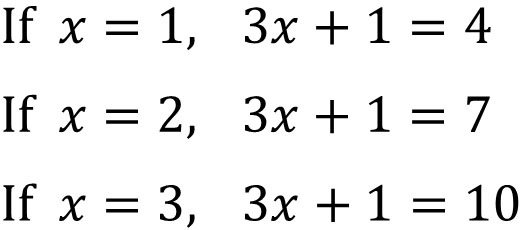It is important that even in algebraic expressions, the answer can be a specific number.

So in an algebraic expression, if the letters are the same, they can be put together by addition or subtraction. In polynomials, terms with the same letters are called like terms (or similar terms). We can distinguish like terms as follows,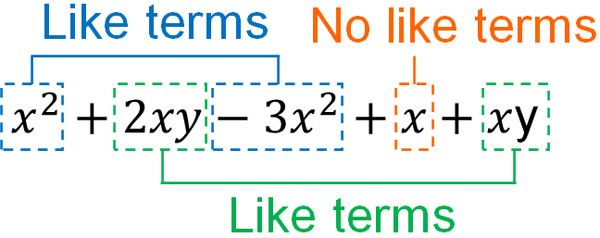If the letters are the same, they are like terms and can be grouped. In other words, you can add or subtract like terms from each other.

Grouping similar terms by adding and subtracting are called combining like terms. To combine like terms, you can do the following.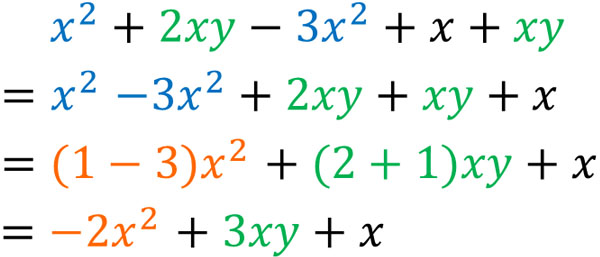After arranging the like terms, add or subtract the coefficients from each other. For example, the coefficient of $x^2$ is 1. And the coefficient of $-3x^2$ is -3. Therefore, the calculation is shown in the above figure.

Once again, it can be written as follows.

• $x^2-3x^2$
• $=(1-3)x^2$
• $=-2x^2$

In addition and subtraction of polynomials, combine like terms. To do this, you need to arrange similar terms. Monomials that have the same letters but different numbers (coefficients) are similar terms.

If the degrees are different, they are not like terms. For example, the following are not similar terms.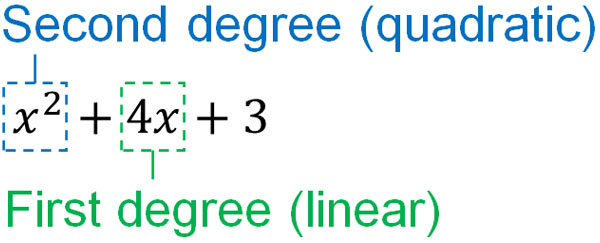Only monomials whose letters are the same, including the degree, are like terms. For example, the following monomials are not similar terms.

• $2x^2$
• $4xy$
• $3a^2$
• $-3x$
• $5y$

Why are these not the like terms? It’s because the letters and degrees are different. Understand that you can only combine like terms when the letters are the same. The reason you can add or subtract like terms is that they have the same properties.

### When Subtracting Polynomials, Be Careful of the Negative

Note that in addition and subtraction of algebraic expressions, we must be especially careful in subtraction. When subtracting polynomials with parentheses, many people make a miscalculation. To be more specific, when there is a minus sign in front of the parentheses, calculation errors occur frequently.

If there is a minus sign in front of the parentheses, the sign changes when the parentheses are removed. For example, the following is an example.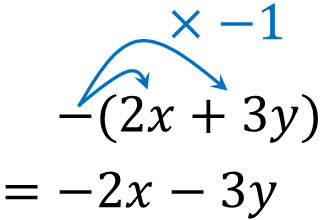In other words, all the signs inside the parentheses change. Why does a minus sign in front of parentheses change all the signs inside the parentheses? The reason is that the number inside the parentheses is considered to be a single number.

In mathematics, there is a rule that parentheses must be calculated first. The reason for this is that even if there are equations inside the parentheses, those equations are considered as a single number. Because it is a single number, it must be calculated first.

For example, if there is the equation $-(1+3)$, how do you calculate it? We can calculate it as follows.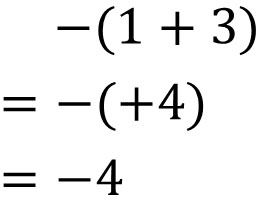In this equation, we do $1+3$ first because of the parentheses. Then, by multiplying by the minus sign, the answer is -4.

On the other hand, if we remove the parentheses first, how can we calculate? In this case, both numbers are multiplied by -1. This means changing all the signs in the parentheses. As a result, the answer is the same as in the previous example, as shown below.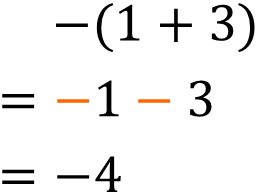For expressions in parentheses, they must be considered the same number. This is why we must change all signs when removing parentheses if there is a minus sign in front of the parentheses. You must not change only one of the numbers in the expression when you remove the parentheses because they are considered the same number.

Therefore, when combining like terms, the calculation is as follows.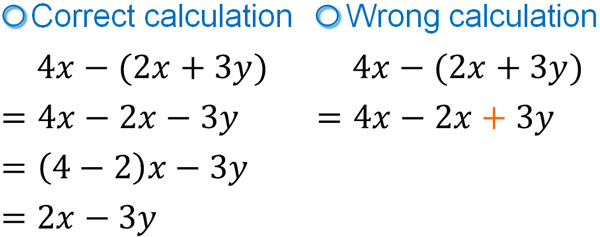When it comes to adding polynomials, we can solve the problem without any difficulty. On the other hand, when subtracting polynomials, many people make mistakes. There are certain parts of polynomial subtraction that can be miscalculated. When you remove the parentheses in subtraction, calculation errors occur frequently.

It is important to understand where calculation errors are most likely to occur. Also, learn how to solve problems correctly. This will help you to avoid miscalculations in the addition and subtraction of polynomials.

## Exercises: Addition and Subtraction of Monomials and Polynomials

Q1: Do the following calculation.

1. $2a÷(-5)$
2. $3b÷2x÷y$

In monomial calculations, you have to convert the division into multiplication. So let’s use the reciprocal and convert all of them into multiplying fractions. Then we get the following.

(a)

$2a÷(-5)$

$=2a×\left(-\displaystyle\frac{1}{5}\right)$

$=-\displaystyle\frac{2a}{5} \left(or-\displaystyle\frac{2}{5}a\right)$

(b)

$3b÷2x÷y$

$=3b×\displaystyle\frac{1}{2x}×\displaystyle\frac{1}{y}$

$=\displaystyle\frac{3b}{2xy} \left(or\displaystyle\frac{3}{2xy}b\right)$

Q2: Do the following calculation.

• $\displaystyle\frac{1}{2}x-\displaystyle\frac{1}{3}x$

You can add or subtract the like terms in algebraic expressions. Of course, fractions and decimals can also be added or subtracted if they are like terms.

$\displaystyle\frac{1}{2}x-\displaystyle\frac{1}{3}x$

$=\displaystyle\frac{3}{6}x-\displaystyle\frac{2}{6}x$

$=\displaystyle\frac{1}{6}x$

Q3: Do the following calculation.

1. $x^2+3-3x-7x^2+5x-10$
2. $(-2x+4y)+(9x-8y)$
3. $(x+3y)-(3x-6y)+4x$

In polynomials, try to combine like terms. This way, you can add and subtract.

If the sign in front of the parentheses is positive, there is nothing to pay attention to. On the other hand, if the sign in front of the parentheses is negative, you will often make a mistake when removing the parentheses. When removing parentheses, it is necessary to change the sign.

(a)

$\textcolor{red}{x^2}+3-\textcolor{blue}{3x}\textcolor{red}{-7x^2}+\textcolor{blue}{5x}-10$

$=\textcolor{red}{x^2- 7x^2}\textcolor{blue}{-3x+5x}+3-10$

$=\textcolor{red}{(1-7)}x^2+\textcolor{blue}{(-3+5)}x+(3-10)$

$=-6x^2+2x-7$

(b)

$(\textcolor{red}{-2x}+\textcolor{blue}{4y)}+(\textcolor{red}{9x}\textcolor{blue}{-8y})$

$=\textcolor{red}{-2x+9x}+\textcolor{blue}{4y-8y}$

$=\textcolor{red}{(-2+9)}x+\textcolor{blue}{(4-8)}y$

$=7x-4y$

(c)

$(x+3y)\textcolor{red}{-(3x-6y)}+4x$

$=x+3y\textcolor{red}{-3x+6y}+4x$

$=x-3x+4x+3y+6y$

$=(1-3+4)x+(3+6)y$

$=2x+9y$

## Learn the Definition and How to Calculate Algebraic Expressions

After studying algebraic expressions in mathematics, almost all calculation exercises will be algebraic expressions. Therefore, if you don’t understand the rules of algebraic expressions, you won’t be able to solve math calculation problems. So be sure to learn the rules of algebraic expressions.

The rules make it possible for us to do the correct calculation. And don’t just remember the rules; learn the reason why.

Note that when adding and subtracting polynomials, it’s important to combine similar terms. Especially if there is a minus sign in front of the parentheses, calculation errors are more likely to occur. Solve the problem while checking whether the plus and minus signs are correct.

Algebraic expressions are important to learn in mathematics. Algebraic expressions can be found in any math problem, so make sure you remember the basics, including the definition of an algebraic expression.